# VBA Last Row or Column with Data

It is important to know how to generate a code that identifies the address of the last row or column with data from a spreadsheet. It is useful as a final counter in Loops and as a reference for intervals.

## VBA Count

The .Count property, when used for a Range object, returns the number of cells in a given range. (whether they are filled or not).

    MsgBox Range("A1:B7").Count 'Returns 14


If you want to know the number of columns or of rows of a given range, you can use the Columns or the Rows properties in conjunction with the Count property.

    MsgBox Range("A1:B7").Rows.Count    'Returns 7
MsgBox Range("A1:B7").Columns.Count 'Returns 2


The .Count property returns the number of objects of a given collection.

If you want to know the number of rows or columns in the worksheet, you can use Rows or Columns without specifying the cells with the Range:

    MsgBox "The number of rows in the worksheet is: " & Rows.Count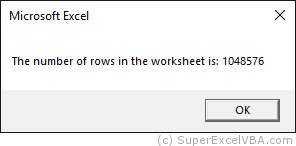MsgBox "The number of columns in the worksheet is: " & Columns.Count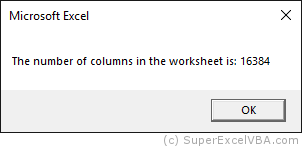## VBA End

Although the Count property is very useful, it returns only the available amount of elements, even if they are without values.

The End property, used in conjunction with the Range object, will return the last cell with content in a given direction. It is equivalent to pressing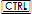+ (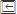or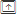or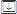or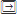).

    Range("A1").End(xlDown).Select
'Range("A1").End([direction]).Select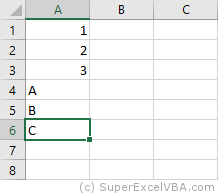When using End it is necessary to define its argument, the direction: xlUp, xlToRight, xlDown, xlToLeft.

You can also use the end of the worksheet as reference, making it easy to use the xlUp argument: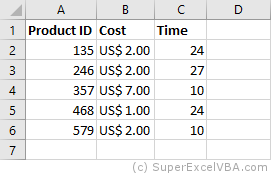MsgBox "The last row with data is number: " & Cells(Rows.Count, 1).End(xlUp).Row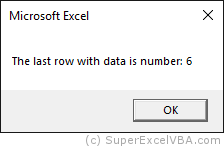## VBA Last Cell Filled

Note that there are some ways to determine the last row or column with data from a spreadsheet:

    Range("A1").End(xlDown).Row
'Determines the last row with data from the first column

Cells(Rows.Count, 1).End(xlUp).Row
'Determines the last row with data from the first column

Range("A1").End(xlToRight).Column
'Determines the last column with data from the first row

Cells(1,Columns.Count).End(xlToLeft).Column
'Determines the last column with data from the first row